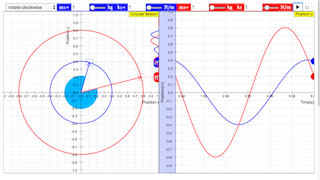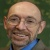Reference Circle

# The Connection between Circular Motion and Simple Harmonic Motion

In this simulation, you can examine the connection between uniform circular motion and simple harmonic motion. This connection is one way to justify the basic equation of motion for an object experiencing simple harmonic motion. x = A cos (ωt) This equation also corresponds to one dimension of uniform circular motion. In this simulation, we even go beyond the equation above a little, and explore the phase angle, φ. In that case, the equation of motion becomes x = A cos (ωt + φ) There are analogous equations for the velocity and the acceleration as a function of time. For more info: http://weelookang.blogspot.sg/2012/07/ejs-open-source-phase-difference-java.html Activities

# Activities

1. When the simulation begins, the two blocks have the same parameters, so they move together. Just focus on the connection between the uniform circular motion (the motion of the ball in a circular path at constant speed) and the simple harmonic motion. Describe the relationship between these two motions.
2. Now, give the two blocks different amplitudes, while keeping everything else the same. How do the motions of the two blocks on springs compare? Can you make sense of this by comparing the harmonic motion to the corresponding circular motion?
3. Set the amplitudes to the same values, but now change either the mass or the spring constant of one of the blocks so that the blocks have different angular frequencies. How do the motions of the two blocks on springs compare? Can you make sense of this by comparing the harmonic motions to the corresponding circular motions?
4. Set the amplitudes, masses, and spring constants of the two blocks so that they match, and now just change the phase of one block with respect to the other. How do the motions of the two blocks on springs compare? Can you make sense of this by comparing the harmonic motions to the corresponding circular motions?
5. What does it mean for two objects to be 180 degrees out of phase?

### Translations

Code Language Translator Run### Software Requirements

SoftwareRequirements

 Android iOS Windows MacOS with best with Chrome Chrome Chrome Chrome support full-screen? Yes. Chrome/Opera No. Firefox/ Samsung Internet Not yet Yes Yes cannot work on some mobile browser that don't understand JavaScript such as..... cannot work on Internet Explorer 9 and below

### CreditsAndrew Duffy; This email address is being protected from spambots. You need JavaScript enabled to view it. remixed based on an eariler applet by andrew duffy; Tan Wei Chiong

[text]

### For Teachers

In this simulation, you can use the combo box on the top left corner to make the circular motion rotate either clockwise or anti-clockwise. The sliders at the top can be adjusted to change the mass (kg) and the spring constant (N/m) of the mass corresponding to the colour.

Research

[text]

[text]

[text]

### end faq

Rating 5.00 (1 Vote)
Parent Category: 02 Newtonian Mechanics
Category: 05 Circle
Hits: 4888